GeeksforGeeks App
Open AppBrowser
Continue

# Python Program to Generate Disk Usage Report in CSV File

In this article, we will see how we could generate disk usage reports and store them in a CSV file using Python using multiple methods.

### Required Modules

We will be using 4 methods here, the modules required would be Shutil, pandas, WMI, OS, and subprocess.

• WMI – WMI is a set of specifications from Microsoft for consolidating the management of devices and applications in a network from Windows computing systems. WMI provides users with data regarding the condition of native or remote pc systems.
• We will use shutil and WMI in different methods, but pandas will be common in both of them, so to install pandas, write the following command in the VScode terminal/ Pycharm terminal or normal Command Prompt.
`pip install pandas`

### Using WMI Module to Generate Disk Usage Report in CSV File

Using the WMI module we can easily fetch the name of the disk, the total size of that disk, and the amount of free space it has (both in bytes). Install the WMI module using the following command:

`pip install WMI`

Here take a variable called key(user can use any name) to initialize the WMI method of the WMI module using which we will get the usage report, then next 3 lines are just 3 blank lists to store the drive name, free_space and total_size of that drive.

## Python3

 `import` `wmi``import` `pandas as pd` `key ``=` `wmi.WMI()``drive_name ``=` `[]``free_space ``=` `[]``total_size ``=` `[]` `for` `drive ``in` `key.Win32_LogicalDisk():``    ``drive_name.append(drive.Caption)``    ``free_space.append(``round``(``int``(drive.FreeSpace)``/``1e``+``9``, ``2``))``    ``total_size.append(``round``(``int``(drive.Size)``/``1e``+``9``, ``2``))``    ``print``(``"================"``)``    ``print``(``"Drive Name :"``, drive.Caption``+``"\n================"``,``          ``"\nFree Space Available in GB : \n"``, ``round``(``              ``int``(drive.FreeSpace)``/``1e``+``9``, ``2``),``          ``"\nTotal Size in GB :\n"``, ``round``(``int``(drive.Size)``/``1e``+``9``, ``2``))` `size_dict ``=` `{``'Directory Name'``: drive_name,``             ``'Free Space (in GB)'``: free_space,``             ``'Total Size (in GB)'``: total_size}` `data_frame ``=` `pd.DataFrame(size_dict)``data_frame.to_csv(``"disk_usage.csv"``)`

Here we are using an iterable drive and using the key variable we are calling the method Win32_LogicalDisk() (Win32_LogicalDisk WMI class represents a data source that resolves to an actual local storage device on a computer system running Windows).

• drive.Caption – Shows the name of the drive.
• drive.FreeSpace – shows the total freespace available in that drive in bytes (That’s why converted to GB by dividing it by 1e+9 and rounding it to 2 decimal places).
• drive.Size – Shows the total size of that drive in bytes ((That’s why converted to GB by dividing it by 1e+9 and rounding it to 2 decimal places).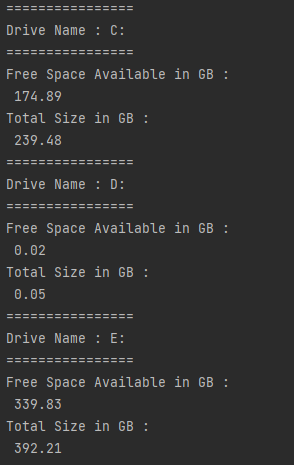Disk usage report using the WMI module

Screenshot of the newly created CSV file: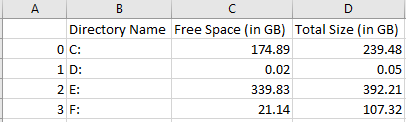### Using the Shutil Module to Generate Disk Usage Report in CSV File

Using the Shutil module we can easily get the total size, used space, and free space of any path provided as an argument to it. Install the Shutil module using the following command.

`pip install pytest-shutil`

## Python3

 `import` `shutil``import` `pandas as pd` `# Path``drive_name ``=` `"C:\\"` `# Get the disk usage statistics``# about the given path``stats ``=` `shutil.disk_usage(drive_name)` `total_size ``=` `round``(stats[``0``]``/``1e``+``9``, ``2``)``free_space ``=` `round``(stats[``2``]``/``1e``+``9``, ``2``)``used_space ``=` `round``(stats[``1``]``/``1e``+``9``, ``2``)` `values ``=` `[drive_name, total_size, free_space, used_space]``index ``=` `[``'Drive Name'``,``         ``'Total Size (in GB)'``, ``'Free Space (in GB)'``,``         ``'Used Space (in GB)'``]` `data_frame ``=` `pd.DataFrame(``list``(``zip``(index, values)),``                          ``columns``=``[``'Information'``, ``'Report'``])``data_frame.to_csv(``"disk_usage.csv"``)` `# Print disk usage statistics``print``(``"Disk usage statistics:"``)``print``(``"Total Size : "``, total_size,``      ``"Free Space Available : "``, free_space,``      ``"Used_Space : "``, used_space)`

Output: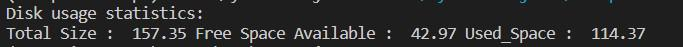Disk usage report using the Sutil module

Here, storing the drive_name first, then passing it as an argument of the method disk_usage() of Shutil module, now using 3 variables we are storing total_size, free_space, and used_space, the disk.usage() method returns the output as a tuple and from that tuple using the index values we are fetching the required ones and converting them to GB from bytes and round them to 2 decimal places. Then using the zip function and two lists we are converting them into a DataFrame and storing it into a CSV file, Also printing the values in the terminal.

The newly created CSV file and its contents are shown below: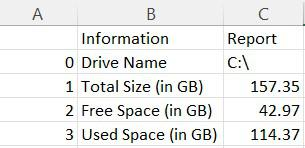Here the sizes are in GB, if the user wants they can change it to MB or anything.

### Using OS Module to Generate Disk Usage Report in CSV File

Using the OS module we can also find out the size of the directory.

## Python3

 `import` `os``import` `pandas as pd`  `def` `get_size(start_path):``    ``total_size ``=` `0``    ``for` `d_path, d_names, f_name ``in` `os.walk(start_path):``        ``for` `f ``in` `f_name:``            ``fp ``=` `os.path.join(d_path, f)``            ``# skip if found as symbolic link``            ``if` `not` `os.path.islink(fp):``                ``total_size ``+``=` `os.path.getsize(fp)` `    ``return` `total_size``/``1e``+``9`  `sizes ``=` `[]``paths ``=` `[]` `# The value of range would be the``# number of drive present in user's device``for` `i ``in` `range``(``2``):``    ``path ``=` `input``(``"Please enter the Path : "``)``    ``paths.append(path)``    ``# Drive paths must have a colon``    ``# and forward slash after their name``    ``sizes.append(get_size(path``+``":\\"``))` `df ``=` `pd.DataFrame({``    ``"Drive Name"``: paths,``    ``"Size of Drive in GB"``: sizes},``    ``# User can give any thing as the index,``    ``# but the amount of them should be same``    ``# as the number of drives passed``    ``index``=``[``1``, ``2``])` `df.to_csv(``"disk_usage_os.csv"``)` `print``(df)`

Output: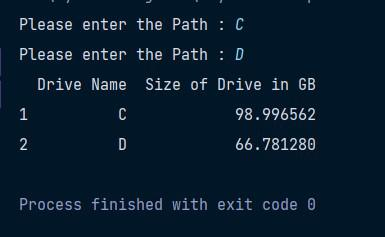Disk usage report using the OS module

Data in the CSV file will be as shown below: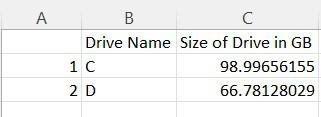### Using the Subprocess Module  to Generate Disk Usage Report in CSV File

Using the subprocess module we can generate the disk usage report and save it as CSV, but this can only be possible if we are using Linux (to save locally) or any online environment like Jupyter Notebook or Google Collab.

If a user encounters anything like a subprocess module not found use the following command to install it:

`pip install subprocess.run`

If the user encounters an error with the pandas too please use the above-mentioned command to install it.

## Python3

 `import` `subprocess``import` `pandas as pd` `process ``=` `subprocess.Popen([``'df'``, ``'-h'``],``                           ``stdout``=``subprocess.PIPE)` `usage ``=` `str``(process.communicate()[``0``]).split(``"\\n"``)` `data ``=` `[]` `for` `i ``in` `range``(``len``(usage)``-``1``):``    ``data.append(usage[i].split())` `index ``=` `data[``0``]` `df ``=` `pd.DataFrame(data, columns``=``index)``data ``=` `df.loc[:, ~df.columns.isin([``'on'``, ``'Mounted'``])]` `data.to_csv(``"disk_usage.csv"``, header``=``False``)``print``(data)`

Output: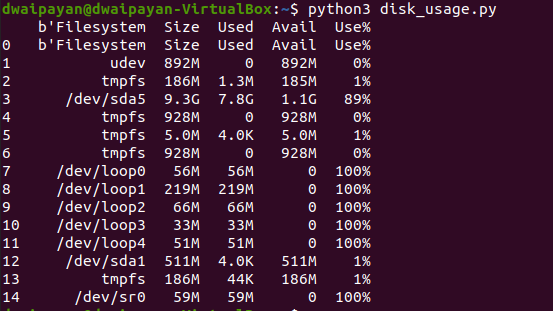Disk usage report using the subprocess module

Data in the CSV file will be as shown below: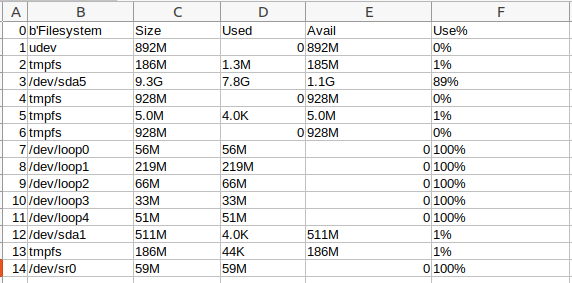#### Using the psutil Module to Generate Disk Usage Report in CSV File

An alternate approach for generating a disk usage report in a CSV file using Python is to use the psutil library. This library provides various functions for retrieving system information, including disk usage.

To use the psutil library, you need to first install it using pip install psutil. Then, you can use the disk_usage function to retrieve the total size, used space, and free space of a given path.

Here is an example of how to use the psutil library to generate a disk usage report in a CSV file:

## Python3

 `import` `psutil``import` `pandas as pd` `# Path to the drive``drive_name ``=` `"C:\\"` `# Get the disk usage statistics``stats ``=` `psutil.disk_usage(drive_name)` `total_size ``=` `stats.total ``/` `1024``*``*``3`  `# Convert bytes to GB``used_space ``=` `stats.used ``/` `1024``*``*``3``free_space ``=` `stats.free ``/` `1024``*``*``3` `# Create a dictionary with the disk usage data``data ``=` `{``'Directory Name'``: drive_name, ``'Total Size (in GB)'``: total_size,``        ``'Used Space (in GB)'``: used_space, ``'Free Space (in GB)'``: free_space}` `import` `pandas as pd` `# Create a Pandas DataFrame with an index``df ``=` `pd.DataFrame(data, index``=``[``0``])` `# Write the DataFrame to a CSV file``df.to_csv(``"disk_usage.csv"``, index``=``False``)` `# Print disk usage statistics``print``(``"Disk usage statistics:"``)``print``(``"Total Size : "``, total_size,``      ``"Free Space Available : "``, free_space,``      ``"Used_Space : "``, used_space)`

This code will generate a CSV file with the following columns: Directory Name, Total Size (in GB), Used Space (in GB), and Free Space (in GB). The values in these columns will correspond to the drive specified in the drive_name variable.

#### Output:

```Disk usage statistics:
Total Size :  237.22753524780273
Free Space Available 57.553279876708984
Used_Space :  179.67425537109375```

My Personal Notes arrow_drop_up